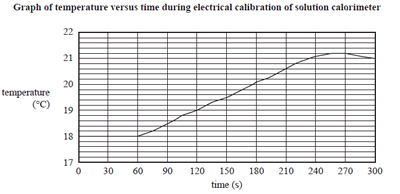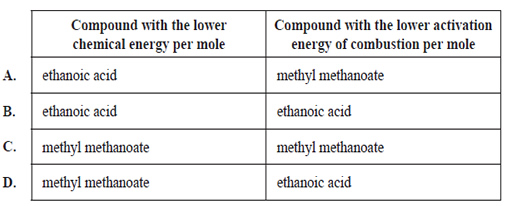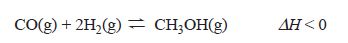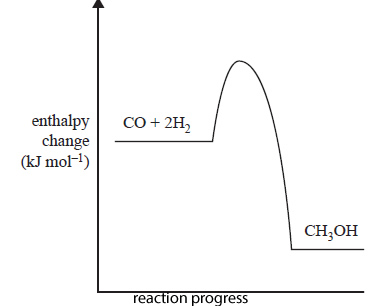Thermochemistry (2020 VCE) 1 )A solution calorimeter containing 350 mL of water was set up. The calorimeter was calibrated electrically and the graph of the results is shown below.a) The calorimeter was calibrated using a current of 2.7 A, starting at 60s. The current was applied for 180 s and the applied voltage was 5.4V. What is the calibration factor for this calorimeter? A. 125 J °C-1 B. 820 J °C-1 C. 847 J °C-1 D. 875 J °C-1 Solution b) This type of calorimeter A. has no heat loss. B. can be used for bomb calorimetry. C. requires electrical calibration in order to determine the calibration factor. D. measures energy changes that can be measured in a bomb calorimeter. Solution2) Which one of the following statements is correct? A. Crude oil can be classified as a biofuel because it originally comes from plants. B. Methane, CH4, can be classified as a fossil fuel because it has major environmental impacts. C. Ethanol, CH3CH2OH, can be classified as a fossil fuel because it can be produced from crude oil. D. Hydrogen, H2, can be classified as a biofuel because, when it combusts, it does not produce carbon dioxide, CO2. Solution3) An experiment was carried out to determine the enthalpy of combustion of propan-1-ol. Combustion of 557 mg of propan-1-ol increased the temperature of 150 g of water from 22.1 °C to 40.6 °C. The enthalpy of combustion is closest to A. −2742 kJ mol-1 B. −1208 kJ mol-1 C. −1250 kJ mol-1 D. −1540 kJ mol-1 Solution4) The combustion of which fuel provides the most energy per 100 g? A. pentane (M = 72 g mol-1), which releases 49,097 MJ tonne B. nitromethane (M = 61 g mol-1, which releases 11.63 kJ g-1 C. butanol (M = 74 g mol-1), which releases 2670 kJ mol-1 D. ethyne (M = 26 g mol-1), which releases 1300 kJ mol-1, Solution5) The heat of combustion of ethanoic acid, C2H4O2, is −876 kJ mol-1 and the heat of combustion of methyl methanoate, C2H4O2, is −973 kJ mol-1. The auto-ignition temperature (the temperature at which a substance will combust in air without a source of ignition) of ethanoic acid is 485 °C and the auto-ignition temperature of methyl methanoate is 449 °C. a) Which one of the following pairs is correct?Solution b) If 0.1 mol of ethanoic acid and 0.1 mol of methyl methanoate were completely combusted in two separate closed vessels under identical conditions, the Maxwell-Boltzmann distribution of the product gases from the combustion of ethanoic acid would be A. broader than the Maxwell-Boltzmann distribution of the methyl methanoate product gases and the chemical energy of the product gases would be identical. B. narrower than the Maxwell-Boltzmann distribution of the methyl methanoate product gases and the chemical energy of the product gases would be identical. C. broader than the Maxwell-Boltzmann distribution of the methyl methanoate product gases and the chemical energy of the ethanoic acid product gases would be higher. D. narrower than the Maxwell-Boltzmann distribution of the methyl methanoate product gases and the chemical energy of the ethanoic acid product gases would be higher. Solution6) Methanol is a very useful fuel. It can be manufactured from biogas. The main reaction in methanol production from biogas is represented by the following equation.This reaction requires the use of a catalyst to maximise the yield of methanol produced in optimum conditions. The energy profile diagram below represents the uncatalysed reaction.On the energy profile diagram above, sketch how the catalyst would alter the reaction pathway Solution7) Methane gas, CH4, can be captured from the breakdown of waste in landfills. CH4 is also a primary component of natural gas. CH4 can be used to produce energy through combustion. a. Write the equation for the incomplete combustion of CH4 to produce carbon monoxide, CO. Solution b. If 20.0 g of CH4 is kept in a 5.0 L sealed container at 25 °C, what would be the pressure in the container? Solution c. A Bunsen burner is used to heat a beaker containing 350.0 g of water. Complete combustion of 0.485 g of CH4 raises the temperature of the water from 20 °C to 32.3 °C. Calculate the percentage of the Bunsen burner’s energy that is lost to the environment. Solution d. Compare the environmental impact of CH4 obtained from landfill to the environmental impact of CH4 obtained from natural gas. Solution